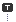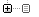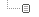# The note of Legendre

to the "Terra incognita"

## Information theory

2010. 5. 5. 21:05

Recently, I read article titled Know When to Stop Designing, Quantitatively. Because first half of article was about mainly information theory which I recently studied in thermal physics, It was very intersting that he is applying this theory into the efficiency issue of user interface.

Introduction of mathematical concepts behind Information theory

These three statements which is belived as a truth about Christmas day.
1. Christmas day falls on a some day of the year.
2. Christmas day falls in the second half of the year.
3. Christmas day falls on the 25th of a month.
Because all days are fall on a some day of the year, the first statement has no new information. The second statement contains more information than first one, at a minimum we know which half of the year the holiday is. The last statement is even more particular and has the most information content.

We can see that more probable statement have less information, If we calculate the probability of the above three statements, the first one will be 1 because it is always true. The second one will be 1/2, and the last one will be 12/365 if we assume that the year is not leap year.

If we add the information above, that can be measured by multiplying two probabilties. Because the first statement has no information, it will be same probability if we multiplying the probability of statements 1 and 2, or 1 and 3. But If we multiplying the probability of statements 2 and 3, the probability will be 6/365.

The probability of two independent true statements are the product of each probabilities. It is obvious to presume that information content can be added. Claude Shannon which is called the father of information theory proposed the definition of information.

Information content  can be calculated using the Q=-k \rm{log} P where P is the probability of the statement and k is some positive constant. Suppose we select \rm{log}_{2}and k =1, then Q is determined in bits.

If we assume W is the number of cases probable, and the probabiltiy of one specific case will be 1/W. So, we can also define the Information content Q = -k log P = -k log (1/W) = -k log (W^(-1)) = -k(-1) log W = k log W.

Various language and Information capacity

In accordance with his calculation, English have approximately 5 bit choice assuming total number of letters is 32. 5 bit choice. Hiragana in Japanese have 76 letters including dakuten and yoon. Katakana has the same number of letters which Hiragana have. and if we use 6 punctuation marks likewise. The number of cases will be 158(76+76+6), and information in bit will be about 7.3 bits. It became Hiragana and Katakana have more information capacity than English letters by 46%. This is not exact, The percentage should be more than that, because I didn't consider Kanji.

In Korean language, one character will be combined using initial, vowel, and final from Jamo in Hangul. The possible number of characters by combinations of Jamo will be 11970. Some of them will be not used in common. Though I ignore 3700 cases of them, the number of bit choice will be more than 13 bit.

There is essential limit of this discussion, because I assume that every language can equally express something or some concept. But it is not true in reality. When we need to introduce new idea that never exists in some cultural envirionment, we have to make additional explanations about that.

It is meaningful in some of senses that the information capacity of one language can be calculated and compared using information as a physical quantity. And it is fun, too., , ,

### 카테고리분류 전체보기 (447)Science (283)ars boni et aequi (55)Routine (83)Language (23)Q&A (1)me2day (1)

### 달력

«   2020/08   »
1
2 3 4 5 6 7 8
9 10 11 12 13 14 15
16 17 18 19 20 21 22
23 24 25 26 27 28 29
30 31